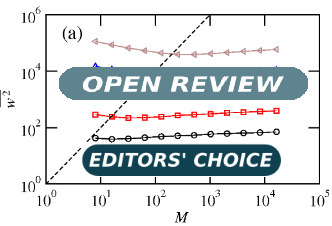# Anisotropic finite-size scaling of an elastic string at the depinning threshold in a random-periodic medium

## Authors

• Sebastián Bustingorry CONICET, Centro Atómico Bariloche, 8400 San Carlos de Bariloche, Río negro, Argentina
• Alejandro B. Kolton CONICET, Centro Atómico Bariloche, 8400 San Carlos de Bariloche, Río negro, Argentina

## Keywords:

Depinning Transition, Disordered Elastic Systems

## Abstract

We numerically study the geometry of a driven elastic string at its sample-dependent depinning threshold in random-periodic media. We find that the anisotropic finite-size scaling of the average square width $\overline{w^2}$ and of its associated probability distribution are both controlled by the ratio $k=M/L^{\zeta_{dep}}$, where $\zeta_{dep}$ is the random-manifold depinning roughness exponent, $L$ is the longitudinal size of the string and $M$ the transverse periodicity of the random medium. The rescaled average square width $\overline{w^2}/L^{2\zeta_{dep}}$ displays a non-trivial single minimum for a finite value of $k$. We show that the initial decrease for small $k$ reflects the crossover at $k \sim 1$ from the random-periodic to the random-manifold roughness. The increase for very large $k$ implies that the increasingly rare critical configurations, accompanying the crossover to Gumbel critical-force statistics, display anomalous roughness properties: a transverse-periodicity scaling in spite that $\overline{w^2} \ll M$, and subleading corrections to the standard random-manifold longitudinal-size scaling. Our results are relevant to understanding the dimensional crossover from interface to particle depinning.

Received: 20 October 2010, Accepted: 1 December 2010; Edited by:  A. Vindigni; Reviewed by: A. A. Fedorenko, CNRS-Lab. de Physique, ENS de Lyon, France; DOI: 10.4279/PIP.0200082010-12-20

## How to Cite

Bustingorry, S., & Kolton, A. B. (2010). Anisotropic finite-size scaling of an elastic string at the depinning threshold in a random-periodic medium. Papers in Physics, 2, 020008. https://doi.org/10.4279/pip.020008

Articles